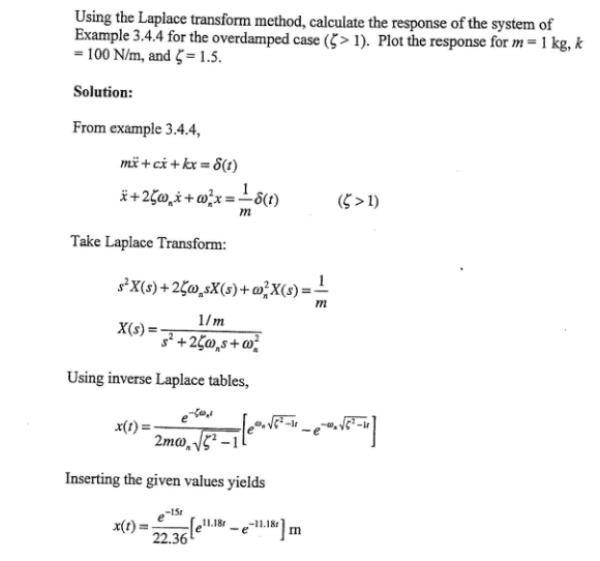Using the Laplace transform method, calculate the response of the system of example for the overdamped case. Plot the response for m=1 kg, k=100 N/m, and z=1.5.Using the Laplace transform method, calculate the response of the system of example for the overdamped case. Plot the response for m=1 kg, k=100 N/m, and z=1.5.

Vibrations Page 1 vibrations vibrations vibrations vibrations vibrations vibrations vibrations Vibrations Page 2